1. How many minutes is it from 23:35 today to 01:15 tomorrow?

A:
B:
C:
D:
E:

2. Which of these is equal to ( 0.1 + 0.2 + 0.3 − 0.4 ) ÷ 0.5 ?

A:
B:
C:
D:
E:

3. Sam has eaten three-quarters of the grapes.

What is the ratio of the number of grapes that remain to the number Sam has eaten?

4. Which of the following five shapes can be cut into four pieces by a single straight cut?

A: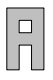B: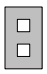C: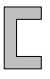D: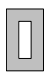E: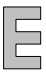5. On Aoife’s 16th birthday, Buster was three times her age. On Aoife’s 21st birthday, how old was Buster?

A:
B:
C:
D:
E:

6. Which of these is closest to 7?

A:
C:
D:

7. The shortest street in the UK, Ebenezer Place in Wick, is 2.06 m long. The Trans-Canada Highway, one of the world’s longest roads, is approximately 7821 km in length.

Approximately, how many times longer than the street is the highway?

8. The diagram shows a kite PGRF inside rhombus PQRS.

Angle PGQ = 35°, angle PFS = 35°, angle PQG = 120° and angle PSF = 120°.

What is the size of angle FPG?

B:
C:
D:
E:

9. What is 50% of 18.3 plus 18.3% of 50?

A:
B:
E:

10. What is the last digit of the smallest positive integer whose digits add to 2019?

A:
B:
C:
D:
E:

11. Two players X and Y take alternate turns in a game, starting with the diagram below:

At each turn, one player writes one of 1, 2 or 3 in an empty circle, so that no two circles connected by an edge contain the same number. A player loses when they cannot go. In each of the five diagrams below it is Y ’s turn.

In which of the diagrams can Y ’s move ensure that X loses the game?

A: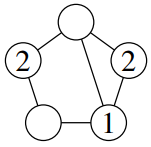B: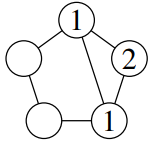C: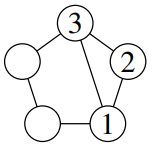D: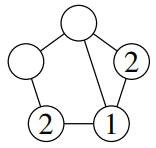E: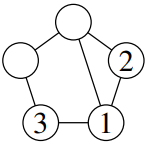12. Jamal writes down a sequence of six integers. The rule he uses is, “after the first three terms, each term is the sum of the three previous terms.” His sequence is —, —, —, 8, 13, 25.

What is his first term?

A:
B:
C:
D:
E:

13. In how many different ways can you spell out JMC, starting at the centre, and moving to the next letter in a neighbouring square – horizontally, vertically, or diagonally – each time?

A:
B:
C:
D:
E:

14. Each edge in the diagram has length 1 cm.

What is the length of the longest path that can be followed along the edges, starting at a vertex and without revisiting any vertex?

A:
B:
C:

15. All four L-shapes shown in the diagram are to be placed in the 4 by 4 grid so that all sixteen cells are covered and there is no overlap. Each piece can be rotated or reflected before being placed and the black dot is visible from both sides.

How many of the 16 cells of the grid could contain the black dot?

A:
B:
C:
D:
E:

Back to the top